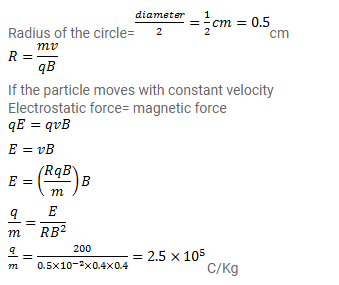# A particle moves in a circle of diameter

Question:

A particle moves in a circle of diameter $1.0 \mathrm{~cm}$ under the action of a magnetic field of $0.40 T$. An electric field of $200 \mathrm{~V} / \mathrm{m}$ makes the path straight. Find the charge/mass ratio of the particle.

Solution: